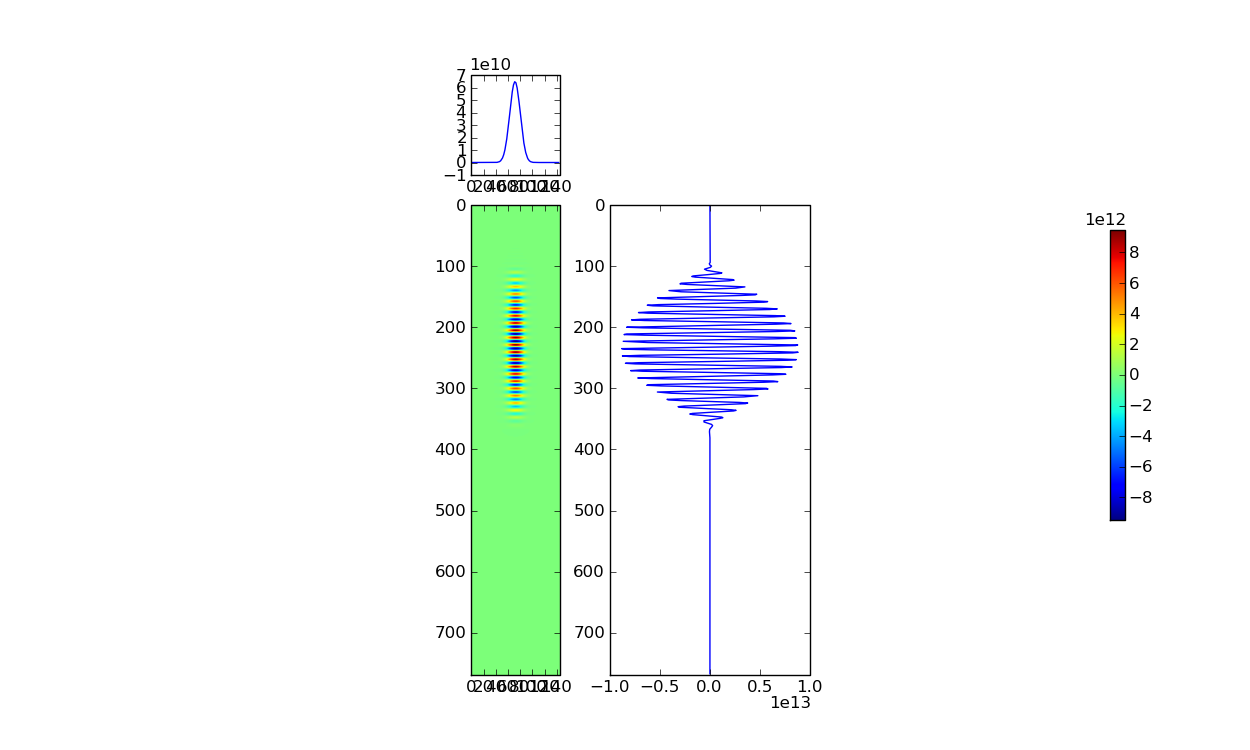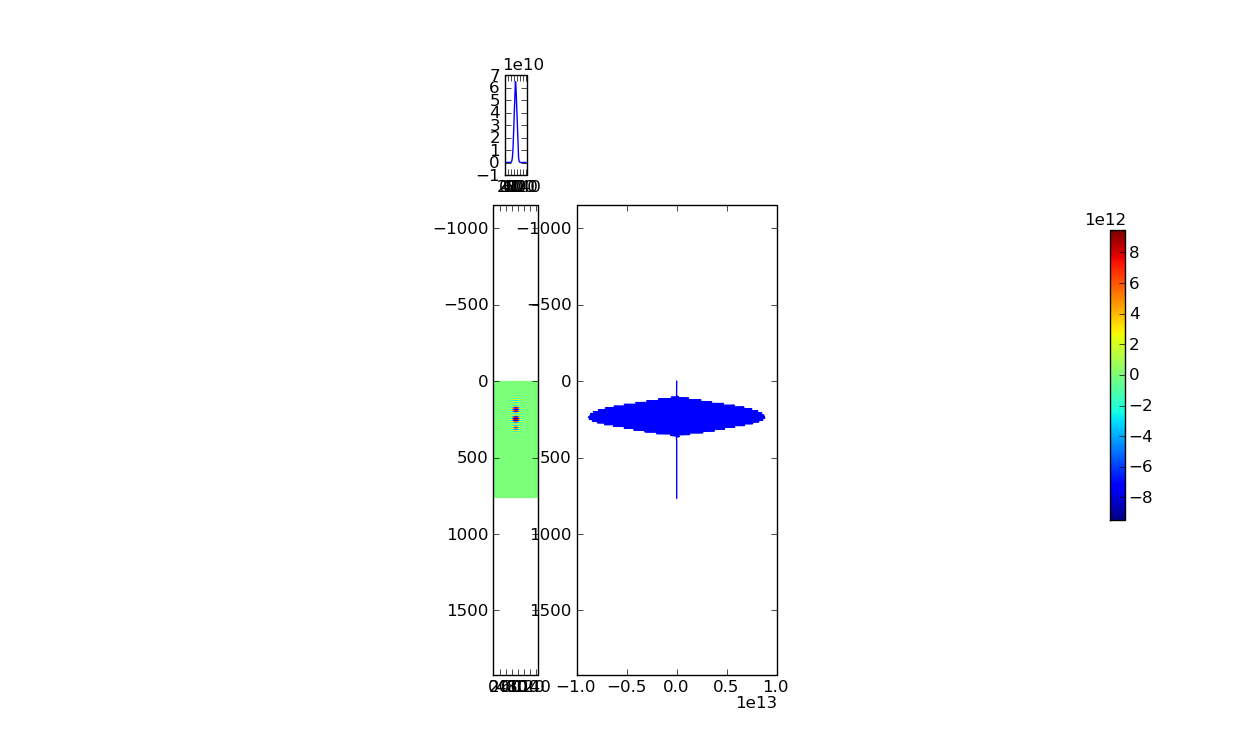# imshow with side plots whitespace problem

Currently, I am trying to plot a 2D array with imshow and two 1D arrays on separate plots attached to the top and right of the imshow image. I got it to work, however when I change the aspect of the image (which I want to do) white space and unusual scalings appear. I want to get rid of it and have the scales that match the aspect.

Basically, I want to do the same thing shown in the example http://matplotlib.sourceforge.net/examples/axes_grid/scatter_hist.html

attached is the result with out the aspect change.

also attached is the result with aspect change attempt.

here is the code that produces the result above:

import numpy as np

import tables

from matplotlib.pyplot import *

import matplotlib as mpl

import matplotlib.cm as cm

fig = figure(figsize=[12.5,7.5])

from mpl_toolkits.axes_grid import make_axes_locatable

#get 3D array from hdf5 file

a = tables.openFile("/Users/magoo/vorpal-data-2/unl-1mm-3d_ElecMultiField_25.h5")

b = a.root.ElecMultiField[ : , : , : ,1]

ax.set_autoscale_on(False)

divider = make_axes_locatable(ax)

#plot line above

axLOutx.plot(b[365,:,75])

axLOutx.set_xlim( (0,145))

#plot line on right

yarr = np.arange(0, np.shape(b[:, 75, 75]), 1)

axLOuty.plot(b[:,75,75], yarr)

axLOuty.set_ylim( (769,0))

# plot image/2D array

im = ax.imshow(b[:,:,75], extent=[0,145,769,0],cmap=cm.jet) # when I add (aspect = .5) as another argument I get what is shown in the second attached image

cb = colorbar(im, fraction=0.015)

plt.draw()

plt.show()The axes_grid toolkit is base on use cases for images of aspect 1, and
I haven't carefully considered cases where the aspect is different
from 1. And I guess this is one of such cases I overlooked.

because of the missing data file, but it works with the the scatter
example you referred).

ax.set_aspect("auto")
divider.set_aspect(True)
divider.get_horizontal()._aspect=0.5

The interface should be improved but I guess this will work.

Regards,

-JJ

···

On Fri, Jul 24, 2009 at 1:19 PM, Jeff Thomas<jeff.thomas137@...287...> wrote:

Currently, I am trying to plot a 2D array with imshow and two 1D arrays
on separate plots attached to the top and right of the imshow image. I got
it to work, however when I change the aspect of the image (which I want to
do) white space and unusual scalings appear. I want to get rid of it and
have the scales that match the aspect.
Basically, I want to do the same thing shown in the
example http://matplotlib.sourceforge.net/examples/axes_grid/scatter_hist.html
attached is the result with out the aspect change.
also attached is the result with aspect change attempt.
here is the code that produces the result above:
import numpy as np
import tables
from matplotlib.pyplot import *
import matplotlib as mpl
import matplotlib.cm as cm

fig = figure(figsize=[12.5,7.5])
from mpl_toolkits.axes_grid import make_axes_locatable
#get 3D array from hdf5 file
a =
tables.openFile("/Users/magoo/vorpal-data-2/unl-1mm-3d_ElecMultiField_25.h5")
b = a.root.ElecMultiField[ : , : , : ,1]
ax.set_autoscale_on(False)
divider = make_axes_locatable(ax)
#plot line above
axLOutx.plot(b[365,:,75])
axLOutx.set_xlim( (0,145))
#plot line on right
yarr = np.arange(0, np.shape(b[:, 75, 75]), 1)
axLOuty.plot(b[:,75,75], yarr)
axLOuty.set_ylim( (769,0))
# plot image/2D array
im = ax.imshow(b[:,:,75], extent=[0,145,769,0],cmap=cm.jet) # when I add
(aspect = .5) as another argument I get what is shown in the second attached
image
cb = colorbar(im, fraction=0.015)

plt.draw()
plt.show()
------------------------------------------------------------------------------

_______________________________________________
Matplotlib-users mailing list
Matplotlib-users@lists.sourceforge.net
https://lists.sourceforge.net/lists/listinfo/matplotlib-users

Jae-Joon Lee,

The data file I worked with is:

for the next 7 days.
It will be removed after Monday, August 3, 2009.

It contains a 4D array which we make into a 3D array. Then that is worked with.

Adding the keyword argument aspect = ‘auto’ works for now. Though, in the future I am not sure this will work for plotting data in different ways. Today I will look into using the divider commands you give.

Thanks,
Jeff Thomas

···

On Sat, Jul 25, 2009 at 2:07 PM, Jae-Joon Lee <lee.j.joon@…287…> wrote:

The axes_grid toolkit is base on use cases for images of aspect 1, and

I haven’t carefully considered cases where the aspect is different

from 1. And I guess this is one of such cases I overlooked.

because of the missing data file, but it works with the the scatter

example you referred).

ax.set_aspect(“auto”)

divider.set_aspect(True)

divider.get_horizontal()._aspect=0.5

The interface should be improved but I guess this will work.

Regards,

-JJ

On Fri, Jul 24, 2009 at 1:19 PM, Jeff Thomas<jeff.thomas137@…287…> wrote:

Currently, I am trying to plot a 2D array with imshow and two 1D arrays

on separate plots attached to the top and right of the imshow image. I got

it to work, however when I change the aspect of the image (which I want to

do) white space and unusual scalings appear. I want to get rid of it and

have the scales that match the aspect.

Basically, I want to do the same thing shown in the

attached is the result with out the aspect change.

also attached is the result with aspect change attempt.

here is the code that produces the result above:

import numpy as np

import tables

from matplotlib.pyplot import *

import matplotlib as mpl

import matplotlib.cm as cm

fig = figure(figsize=[12.5,7.5])

from mpl_toolkits.axes_grid import make_axes_locatable

#get 3D array from hdf5 file

a =

tables.openFile("/Users/magoo/vorpal-data-2/unl-1mm-3d_ElecMultiField_25.h5")

b = a.root.ElecMultiField[ : , : , : ,1]

ax.set_autoscale_on(False)

divider = make_axes_locatable(ax)

#plot line above

axLOutx.plot(b[365,:,75])

axLOutx.set_xlim( (0,145))

#plot line on right

yarr = np.arange(0, np.shape(b[:, 75, 75]), 1)

axLOuty.plot(b[:,75,75], yarr)

axLOuty.set_ylim( (769,0))

# plot image/2D array

im = ax.imshow(b[:,:,75], extent=[0,145,769,0],cmap=cm.jet) # when I add

(aspect = .5) as another argument I get what is shown in the second attached

image

cb = colorbar(im, fraction=0.015)

plt.draw()

plt.show()

Matplotlib-users mailing list

Matplotlib-users@lists.sourceforge.net

https://lists.sourceforge.net/lists/listinfo/matplotlib-users